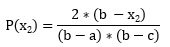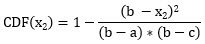# “Easy” Excel Inverse Triangular Distribution for Monte Carlo Simulations Redux

Back in the dark ages when access to computers was not all that common, I was faced with developing a project schedule for, to me, a complex construction project. I was not that long out of school, so I sought out my boss with the hope he would give me some guidance on how to approach the problem.

He told me to use three-point estimation and to talk to some of the older engineers in the firm to get their ideas on the likely outcomes. So, I did and learned that the three points he was talking about were the worst case, the best case, and the most likely case for what would happen during the project. (Wikipedia, n.d.)

He also directed me to consider using PERT. I did and learned that form of project management scheduling including consideration of the optimistic time estimate (o), the most likely or normal time estimate (m), and the pessimistic time estimate (p). In PERT, instead of using probabilities for each estimate of the time required, the task time is calculated as (o + 4m + p) ÷ 6. (Taylor Jr., 2011)

To model a three-point estimate with a probability distribution you need to use a triangular distribution. Today, three-point estimates are commonly used in business and engineering, so it is somewhat surprising that Excel does not have a built-in function to help. I was recently faced with this dilemma in my quantitative methods course which I am trying to migrate away from expensive software solutions.

We were working on modeling business problems using the Monte Carlo method. In the example “Make vs Outsource” problem I wanted to use, the demand for the new SSD (solid state drive) in our case study was forecast to have a worst case, best case, and most likely value.

### This is the basic model:

How could we model this demand growth as a random variable using a triangular distribution for use in a Monte Carlo simulation?

As you begin to look at a triangular distribution, there is nothing more than basic geometry and algebra required.

In the image above, a is the minimum value, b is the maximum value, and c is the most likely value, the mode. The probability distribution represented by the area in the larger triangle is continuous and, of course, equal to 1.

Recall the area of a triangle is ½ * base * height. Since the area = 1, 1 = ½ * (b-a) * h. Rearranging, we get

h = 2/(b-a).

Looking at the smaller yellow triangle, A1, that area would represent the cumulative probability distribution of x > a and x <= c, the most likely value.

P(a < x <= c), then, is equal to ½ * (c-a)*h = ½ * (c-a) * [2/(b-a)], or, with a bit of rearranging,

P(a < x <=c) = (c-a)/(b-a).

If we set a < x < b, we can then say that the cumulative probability x <= c, the most likely value,

P(<=c) = (c-a)/(b-a).

### Let’s look at an example of three-point forecast increases in demand for a product:

Worst case increase = 2%; best case increase = 10%, and most likely case increase = 7%.

Thus, a = 2%, b = 10%, and c = 7%. The probability x being less than or equal to the most likely value for demand increase of 7% is
P(<=c) = (7-2)/(10-2) =  5/8 = 0.625.

Let’s look at a more general case.

For the case of x1, a < x1 < = c,For x2, c < x2 < = b,(Weissteain, n.d.)

And again, by some algebraic manipulation, we can find the cumulative probability distributions:(Weissteain, n.d.)

So, these two equations will give the probability for an x for a triangular distribution, but how do we use them in a Monte Carlo simulation where we want to randomize the x values for a triangular distribution?

For variables that follow a normal distribution, we can use the Excel RAND function to generate probabilities and, with the NORM.INVERSE, to then generate random values of x (see image 1 for an example). So, to generate random values of x that follow a triangular distribution, we need to develop an inverse of the two CDF formulas above.

To do that, we can generate random probabilities (P1 and P2) using the RAND() function and then set them equal to the CDF for each of the two equations. Then use algebra to solve for x for each of the two cases.

For the first part:

 P1 = (x1-a)^2/((b-a)*(c-a)) P1*((b-a)*(c-a)) = (x1-a)^2 SQRT(P1*(b-a)*(c-a)) = x1-a x1 = a +SQRT(P1*(b-a)*(c-a)) For the second part: P2 = 1 – (b-x2)^2/((b-a)*(b-c)) P2*(b-a)*(b-c) = 1*(b-a)*(b-c)-(b-x2)^2 (b-x2)^2 = 1*(b-a)*(b-c) – P2*(b-a)*(b-c) (b-x2)^2 = (1-P2)*(b-a)*(b-c) (b-x2) = SQRT((1-P2)*(b-a)*(b-c)) -x2 = SQRT((1-P2)*(b-a)*(b-c))-b x2 = b-SQRT((1-P2)*(b-a)*(b-c))

Now, put them into Excel format and use an IF statement to pick the one to use. To do that we will use the cumulative probability that x = c as the decision point.

That is P(c ) = (c-a)/(b-a)

If P(x) <= P(c ), use the equation for x1, else, use the equation for x2.

Here is the implementation in Excel. I then link cell B6 into the Make vs Buy model for the demand and conduct the Monte Carlo simulation. the red and blue colors refer back to the two equations developed above for x1 and x2.

#### To see how this works, I ran a 5000 trial simulation and plotted a histogram of the x-values generated.I think that is pretty good. And all done using basic Excel – no expensive add-ins.

Triangular_Distribution_X-value_Calculator_V1.00_Dawn_Wright_PhD

Petty, N., & Dye, S. (2013, June 11). Triangular Distributions. Retrieved from Statistics Learning Center: https://learnandteachstatistics.files.wordpress.com/2013/07/notes-on-triangle-distributions.pdf

Taylor Jr., J. (2011, July 6). The History of Project Management: Late 20th Century Process and Improvements. Retrieved from Bright Hub Project Management: http://www.brighthubpm.com/methods-strategies/11663-late-20th-century-process-and-improvements-in-pm-history/

Weissteain, R. (n.d.). Triangular Distribution. Retrieved from Wolfram Mathworld – A Worfram Web Resource: http://mathworld.wolfram.com/TriangularDistribution.html

### 19 thoughts on ““Easy” Excel Inverse Triangular Distribution for Monte Carlo Simulations Redux”

1.Thank you for the clear instructions and assistance. This was exactly what I needed to complete my own Monte Carlo simulations.

2.Dr. Wright,
Thank you for publishing this article and downloadable spreadsheet. In geology we deal with many skewed distributions with limited samples and we are forced to make estimates of min, max and most likely cases. Your Excel method is a real gem!

1.Thanks for the positive feedback.

3.this is super useful! one scenario it may not work for is what if you have negative numbers? for example growth is uncertain between -10% to 5%?

4.Great work Dawn. Thank you

5.Thank you for this clear explanation and generous sharing of your work; this is amazing!

6.Fantastic, thanks for sharing

7.Thanks for the great article and the very throughout explanation, I got a little lost in the algebra though :s

8.Dr.Wright ! This is very useful. Just to add on.

If anyone stumbled onto this page like me looking for a way to model BetaPERT distributions (Which are similar to Triangular distributions in that they accept 3 point min-mode-max inputs) , you can do this directly in Excel via the BETA.INV function

Assuming Min =Cell A1 ; Mode = Cell B1 ; Max = Cell C1, you need to first ‘reverse calculate’ the alpha , beta , mean variables

mean =(A1+4*B1+C1)/6
alpha =((mean-A1)*(2*B1-A1-C1))/((B1-Mean)*(C1-A1))
beta = =(alpha*(C1-mean))/(mean-A1)

…then to generate a beta distribution you use the formula = =BETA.INV(RAND(),alpha,beta,min,max)

Be aware though, the “randomness” of the distribution from the RAND() function may not perfect, I remember reading a paper criticizing (an older version of) Excel’s implementation of the Wichman-Hill random number generation (As opposed to using better techniques like a Mersenne Twister etc)

Now a trickier question I have for you or anyone else reading – Has anyone figured out how to “induce correlation” between samples of variables in plain vanilla Excel ? (I.e In commercial add-ins like Palisade’s @Risk or Decisioneering’s Crystal Ball , they use an Iman Conover Method so you can directly specify how strongly correlated various random variables will be when sampling and even alert you when you create an inconsistent set of relationships )

9.Thank you. This helps me a lot

10.Thank you very much for this post. This really helps me a lot!

11.Amir Abd-Ellatif

Thank you so much for this post, any chance we could download the excel file i tried the link did not work

1.Please try again. I reset my site optimizer and it should allow you to download the file now. If not, email me doc@drdawnwright.com.

12.13.Good point. I will dig into this.

14.Could I integrate the rand() function directly in the formula for x in B6 and drop B5?

15.It is also possible to define the probability once and name it as a variable that can be used multiple times in the formula. This is particularly useful when more than one triangular distribution needs to be calculated and each one requires its own probability.

= LET(P_x, RAND(),
IF(P_x<=(B3-B2)/(B4-B2),B2+SQRT(P_x*(B4-B2)*(B3-B2)),B4-SQRT((1-P_x)*(B4-B2)*(-B3+B4)))
)

16.Hi,
I am doctor trying to see possible outcomes in the patients transfer from hospital to the Intermediate Care Centres based on their risk score ( Barthel Scale), length of stay and level of care which is expressed through Barthel scale ( Low, Medium, High)
We want to discharge Medically Fit For Discharge occupying 80 out of 300 beds nearly every day ( I have data of possible discharge for every day for 4 months).
I can explain better to somebody ready to help, please. If you want email me with replay as well as chat, please.

17.Thank you for the clear explanation and model. It is very helpful to be able to do this in native Excel without using VBA. Would it be possible to expand the function so that instead of using min and max, to use a value that represented the 10 percentile value on the low end and the 90 percentile value on the high end as inputs along with the most likely? My experience in Monte Carlo forecasts tells me that people are better at estimating a “bad” value then they are able to estimate the “worst”.

This site uses Akismet to reduce spam. Learn how your comment data is processed.